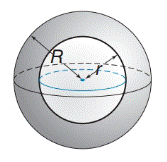Chapter 9.4, Problem 50E### Elementary Geometry for College St...

6th Edition
Daniel C. Alexander + 1 other
ISBN: 9781285195698

#### Solutions

Chapter
Section### Elementary Geometry for College St...

6th Edition
Daniel C. Alexander + 1 other
ISBN: 9781285195698
Textbook Problem
14 views

# Derive a formula for the total surface area of the hollow-core sphere.(Note: Include both interior and exterior surface areas.)To determine

To find:

The formula for the total surface area of the hollow-core sphere.

Explanation

Approach:

A sphere is a three dimensional solid figure, which is made up of all the points in space, which lie at a common distance, called the radius, from a fixed point called the center of the sphere. The surface area of a sphere S=4πr2, where r is the radius of the sphere.

All solid bodies occupy space. The measure of occupied space is called the volume of the object. Volume of a sphere is V=43πr3.

Concentric spheres have the same center but different radii.

Calculation:

Consider a hollow-core sphere.

Length of the radius of the inside sphere =r

Length of the radius of the outside sphere =R.

To find the total surface area of the hollow-core sphere, we calculate the surface area of both the concentric spheres and add them up.

Total surface area of hollow-core sphere =Surface area o

### Still sussing out bartleby?

Check out a sample textbook solution.

See a sample solution

#### The Solution to Your Study Problems

Bartleby provides explanations to thousands of textbook problems written by our experts, many with advanced degrees!

Get Started

#### In Exercises 914, evaluate the expression. 9. (94)3/2

Applied Calculus for the Managerial, Life, and Social Sciences: A Brief Approach

#### Describe the purpose of a dismantling design.

Research Methods for the Behavioral Sciences (MindTap Course List)

#### Which graph represents y=x+1x

Study Guide for Stewart's Single Variable Calculus: Early Transcendentals, 8th

#### Draw a scatterplot for which r = 1.

Introduction To Statistics And Data Analysis